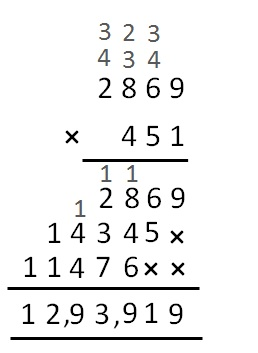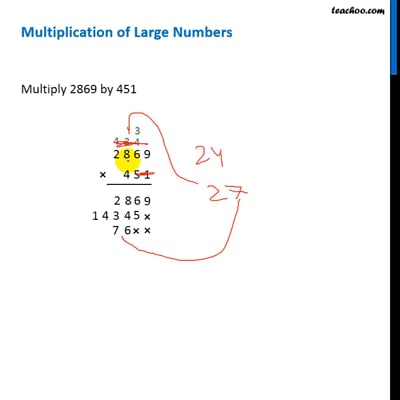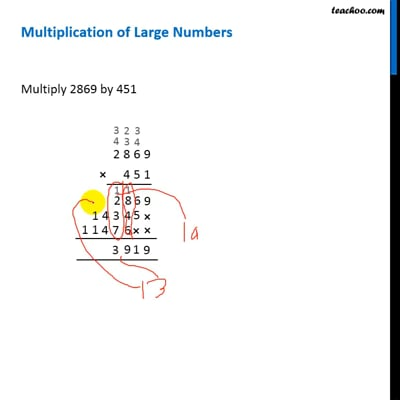Multiplication of large numbers

Chapter 1 Class 6 Knowing our Numbers
Concept wise

## Multiplication of Large Numbers

Multiply 2869 by 451So, multiplication is 12,93,919This video is only available for Teachoo black usersThis video is only available for Teachoo black users

Introducing your new favourite teacher - Teachoo Black, at only ₹83 per month

### Transcript

Multiplication of Large Numbers Suppose we want to multiply 2869 by 451 We follow these steps 1. We write the numbers on top of each other (one digit below the other) 2. We multiply the digit on the below number, to the digits on the number on top. going from right to left. 3. First we multiply 1 with 2869. 4. Multiplying 1 with 9, we get 9. We put 9 below. 5. Multiplying 1 with 6, we get 6. We put 6 below. 6. Multiplying 1 with 8, we get 8. We put 8 below. 7. Multiplying 1 with 2, we get 2. We put 2 below. 8. Now, we multiply 5 with 2869. But before multiplying, we put an x below the first digit on the right. 9. Multiplying 5 with 9, we get 45. We put last digit 5 below 6, the first digit 4 on the top number, above 6. 10. Now, multiplying 5 with 6, we get 30, adding the 4 on top, we get 34. Putting last digit 4 below, and first digit 3 on top of next digit 8 11. And so we repeat our process and get our multiplication So, multiplication is 12,93,919